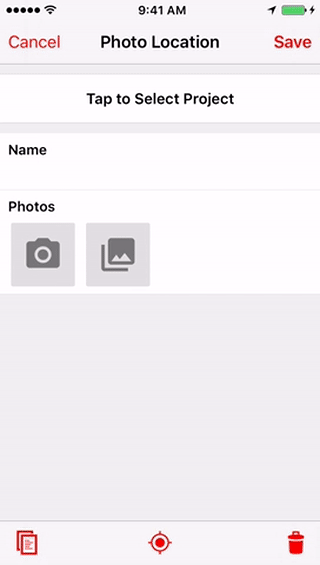# Compare photo location to record location

This example demonstrates how to compare the geotagged location of an attached photo with the location of the record to alert the user if there may be an issue. NOTE: The LATITUDE and LONGITUDE functions will only return values if a record's geometry type is set to `Point`, and there is a location set. Unless you have enabled Lines and Polygons for your app, the `Point` type will be the only available geometry type for records in the app.``````// source: https://www.geodatasource.com/developers/javascript
function findDistance(lat1, lon1, lat2, lon2, unit) {
var radlat1 = Math.PI * lat1 / 180;
var radlat2 = Math.PI * lat2 / 180;
var theta = lon1 - lon2;
var radtheta = Math.PI * theta / 180;
dist = Math.acos(dist);
dist = dist * 180 / Math.PI;
dist = dist * 60 * 1.1515;
if (unit == "K") {
dist = dist * 1.609344;
}
if (unit == "N") {
dist = dist * 0.8684;
}
return dist;
}

function validateDistance(event) {
if (event.value.latitude && event.value.longitude) {
var kilometers = findDistance(event.value.latitude, event.value.longitude, LATITUDE(), LONGITUDE(), 'K');
var meters = Math.round(kilometers * 1000);
if (meters > 20) {
ALERT('This photo is over 20 meters from the record location. (' + meters + ' meters)');
}
}
}